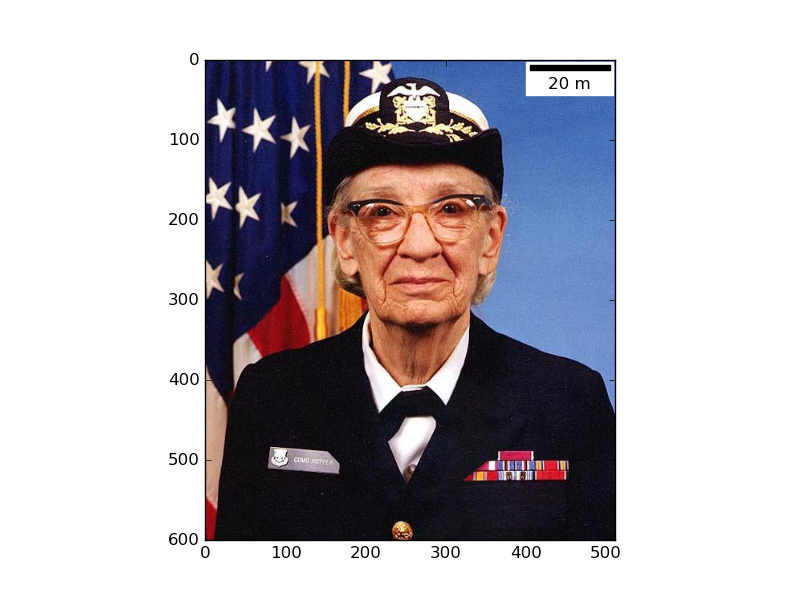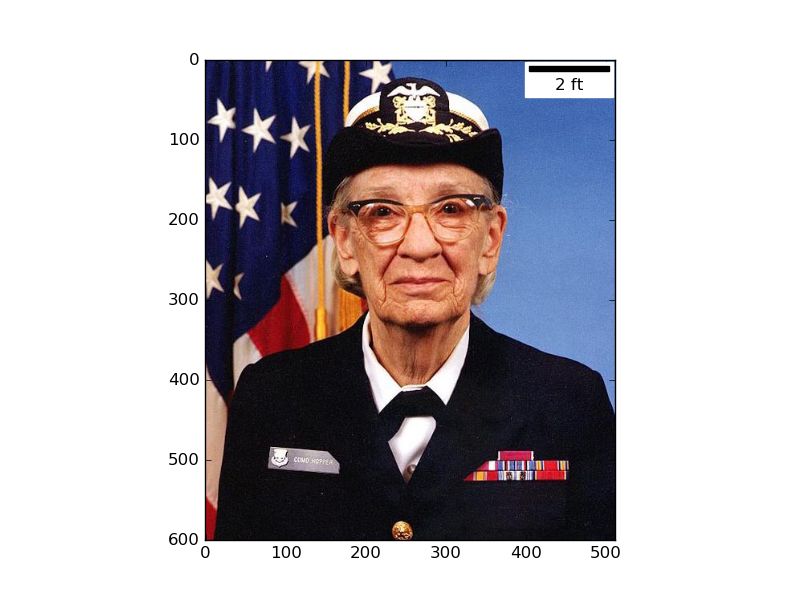# ppinard / matplotlib-scalebar

Provides a new artist for matplotlib to display a scale bar, aka micron bar.
Python

## Latest commitFetching latest commit…
Cannot retrieve the latest commit at this time.

## Files

Type Name Latest commit message Commit time
Failed to load latest commit information.docmatplotlib_scalebar.coveragerc May 15, 2016.gitattributes.gitignore.project.pydevproject.sample.travis.ymlLICENSEMANIFEST.inREADME.rstrequirements.txtsetup.cfgsetup.pyversioneer.py

# matplotlib-scalebarProvides a new artist for matplotlib to display a scale bar, aka micron bar. It is particularly useful when displaying calibrated images plotted using plt.imshow(...).The artist supports customization either directly from the ScaleBar object or from the matplotlibrc.

## Installation

Easiest way to install using `pip`:

```\$ pip install matplotlib-scalebar
```

For development installation from the git repository:

```\$ git clone git@github.com:ppinard/matplotlib-scalebar.git
\$ pip install -e matplotlib-scalebar
```

## How to use

There are two modes of operation:

1. Length, value and units of the scale bar are automatically determined based on the specified pixel size dx and length_fraction. The value will only take the following numbers: 1, 2, 5, 10, 15, 20, 25, 50, 75, 100, 125, 150, 200, 500 or 750.
2. The desired value and units are specified by the user (fixed_value and fixed_units) and the length is calculated based on the specified pixel size dx.

The constructor arguments dx and units specify the pixel dimension. For example `scalebar = ScaleBar(0.2, 'um')` indicates that each pixel is equal to 0.2 micrometer. If the the axes image has already been calibrated by setting its `extent`, set dx to 1.0.

Special notes for geospatial plots: If you are plotting geospatial coordinates (such as scatterplots of the location of structures, geopandas geodataframe plots, etc.), `dx` needs to be set differently depending on the coordinate system:

• For UTM based coordinate system, where the X and Y are in meters, simply set `dx = 1`.
• For WGS or NAD based coordinate system, where X and Y are in latitude (Y) and longitude (X), compute the distance between two points at the latitude (Y) you wish to have the scale represented and are also one full degree of longitude (X) apart, in meters. For example `dx = great_circle_distance((X, Y), (X + 1, Y))`

The system of units (SI, imperial, etc.) is defined by the argument dimension. By default, the scale bar uses SI units of length (e.g. m, cm, um, km, etc.). See examples below for other system of units.

## Example

Here is an example how to add a scale bar:

```>>> import matplotlib.pyplot as plt
>>> import matplotlib.cbook as cbook
>>> from matplotlib_scalebar.scalebar import ScaleBar
>>> plt.figure()
>>> plt.imshow(image)
>>> scalebar = ScaleBar(0.2) # 1 pixel = 0.2 meter
>>> plt.show()
```

The scale bar also works with reciprocal units,:

```>>> from matplotlib_scalebar.scalebar import SI_LENGTH_RECIPROCAL
>>> scalebar = ScaleBar(0.2, '1/cm', SI_LENGTH_RECIPROCAL) # 1 pixel = 0.2 1/cm
```

imperial units:

```>>> from matplotlib_scalebar.scalebar import IMPERIAL_LENGTH
>>> scalebar = ScaleBar(0.2, 'ft', IMPERIAL_LENGTH) # 1 pixel = 0.2 feet
```and system defined by the Dimension class.

## ScaleBar arguments

Here are parameters of the ScaleBar class constructor.

• `dx`: Size of one pixel in units specified by the next argument (required). Set `dx` to 1.0 if the axes image has already been calibrated by setting its `extent`.
• `units`: units of dx (default: `m`)
• `dimension`: dimension of dx and units. It can either be equal
• `si-length`: scale bar showing km, m, cm, etc.
• `imperial-length`: scale bar showing in, ft, yd, mi, etc.
• `si-length-reciprocal`: scale bar showing 1/m, 1/cm, etc.
• `pixel-length`: scale bar showing px, kpx, Mpx, etc.
• `angle`: scale bar showing °, ʹ (minute of arc) or ʹʹ (second of arc).
• a `matplotlib_scalebar.dimension._Dimension` object
• `label`: optional label associated with the scale bar (default: `None`, no label is shown)
• `length_fraction`: length of the scale bar as a fraction of the axes's width (default: `rcParams['scalebar.lenght_fraction']` or `0.2`)
• `height_fraction`: height of the scale bar as a fraction of the axes's height (default: `rcParams['scalebar.height_fraction']` or `0.01`)
• `location`: a location code (same as legend) (default: `rcParams['scalebar.location']` or `upper right`)
• `pad`: fraction of the font size (default: `rcParams['scalebar.pad']` or `0.2`)
• `border_pad`: fraction of the font size (default: `rcParams['scalebar.border_pad']` or `0.1`)
• `sep`: separation between scale bar and label in points (default: `rcParams['scalebar.sep']` or `5`)
• `frameon`: if `True`, will draw a box around the scale bar and label (default: `rcParams['scalebar.frameon']` or `True`)
• `color`: color for the scale bar and label (default: `rcParams['scalebar.color']` or `k`)
• `box_color`: color of the box (if frameon) (default: `rcParams['scalebar.box_color']` or `w`)
• `box_alpha`: transparency of box (default: `rcParams['scalebar.box_alpha']` or `1.0`)
• `scale_loc`: either `bottom`, `top`, `left`, `right` (default: `rcParams['scalebar.scale_loc']` or `bottom`)
• `label_loc`: either `bottom`, `top`, `left`, `right` (default: `rcParams['scalebar.label_loc']` or `top`)
• `font_properties`: font properties of the label text, specified either as dict or fontconfig pattern (XML).
• `label_formatter`: custom function called to format the scalebar text. Needs to take 2 arguments - the scale value and the unit. (default: `None` which results in `<value> <unit>`)
• `fixed_value`: value for the scale bar. If `None`, the value is automatically determined based on length_fraction.
• `fixed_units`: units of the fixed_value. If `None` and fixed_value is not `None`, the units of dx are used.
• `animated`: animation state (default: `False`)

## matplotlibrc parameters

Here are parameters that can be customized in the matplotlibrc file.

• `scalebar.length_fraction`: length of the scale bar as a fraction of the axes's width (default: `0.2`)
• `scalebar.height_fraction`: height of the scale bar as a fraction of the axes's height (default: `0.01`)
• `scalebar.location`: a location code (same as legend) (default: `upper right`)
• `scalebar.pad`: fraction of the font size (default: `0.2`)
• `scalebar.border_pad`: fraction of the font size (default: `0.1`)
• `scalebar.sep`: separation between scale bar and label in points (default: `5`)
• `scalebar.frameon`: if True, will draw a box around the scale bar and label (default: `True`)
• `scalebar.color`: color for the scale bar and label (default: `k`)
• `scalebar.box_color`: color of the box (if frameon) (default: `w`)
• `scalebar.box_alpha`: transparency of box (default: `1.0`)
• `scalebar.label_top`: if True, the label will be over the scale bar (default: `False`)
• `scalebar.font_properties`: font properties of the label text, specified either as dict or fontconfig pattern (XML).

## Release notes

### 0.6.0

• Add blit support and fix documentation (PR#22)
• Fix issue with getting the wrong preferred values for the scale bar. (PR#23)
• Package LICENSE file to distribution. (PR#24)

### 0.5.1

• Remove leftover print statement (#18)

### 0.5.0

• Display micro symbol in text mode (#15)
• Fix error in length of scale bar (#14). The bar was drawn with an edge around it which made it longer than the actual size.

### 0.4.1

• Fix deprecated usage of is_string_like (#11)

### 0.4.0

• Add possibility to specified a fixed value for the scale bar (#9)